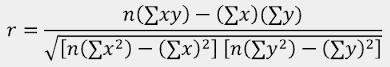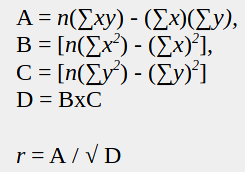Basic UVM testbench for a Stream Processor
next section →

### Doing the math2 for Pearson's CoefficientThe highest value required for the Pearson's Correlation Coefficient (an intermediate value in the calculation) is
Nmax2 * Xmax x Ymax, or 11 * 11 * 126 * 126 = 1,920,996.
That fits into 21 bits (221=2,097,152).

By doing an 8-bit left shift prior to division, now requiring 29-bit registers, I can let Verilog just truncate the fractional part.

Using one byte for the correlation coefficient, b.bbbbbb, is enough to represent a value down to +/- 2-7, or +/-0.0078125.

There is probably no reason to make pearsons_r out of 29-bit registers, so they'll be 32-bit wide.

The calculation can be broken down into the form:and the intermediate values A, B, C, and D only require large interger storage. The squareroot and the division will require division, resulting in a fractional component. If I do an 8-bit left shift prior to division, now requiring 29-bit registers, I can let Verilog just truncate the fractional part of the division (I'm sure the technical name for this is fractional chucking). No floating point math is required.

Using one byte for the correlation coefficient, b.bbbbbb, is enough to represent a value down to +/- 2-7, or +/-0.0078125.

There is probably no reason to make the pearsons_r RTL out of 29-bit registers, so they'll be 32-bit wide.

But ... the hardware is not an efficient implementation as of yet. The diagram on this page A specific Device Under Test shows a fixed multiplication by N from the beginning, meaning that the intermediate values for the denominator are not in step with the sample number, but also that the denominator value far exceeds the 32 bit range. It takes a while for the sumX*sumX and sumY*sumY terms to catch up to the N*sumXsquared terms.
I have used 40 bit registers throughout and will revisit this properly when it is time to convert the code to a synthesizable implementation.

This is a work in progress